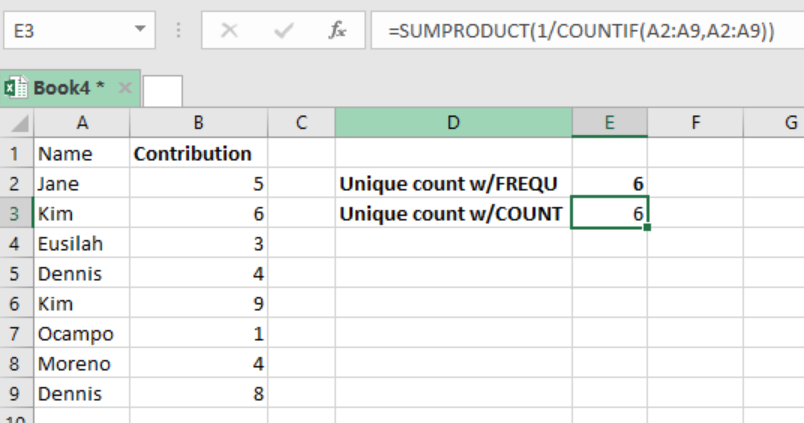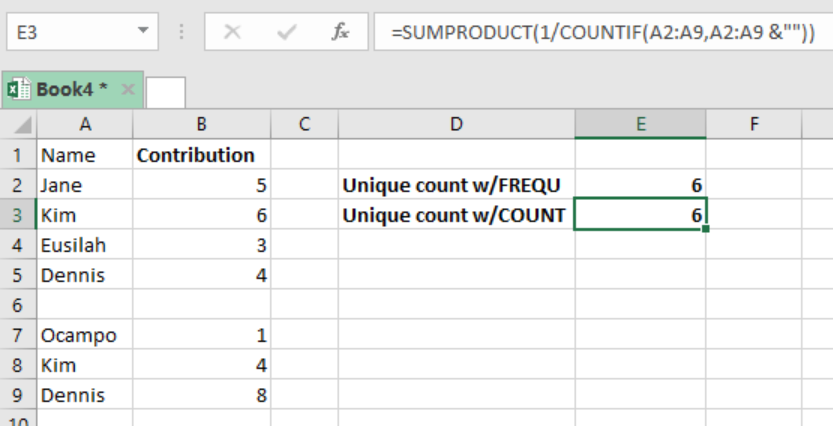Get instant live expert help with Excel or Google Sheets“My Excelchat expert helped me in less than 20 minutes, saving me what would have been 5 hours of work!”

#### Post your problem and you’ll get Expert help in seconds.

Your message must be at least 40 characters
Our professional Expert are available now. Your privacy is guaranteed.

# How to Count Unique Values in a Range with COUNTIF

To count the number of unique values in a range of cells in excel, we need to use a formula that is based on the COUNTIF as well as the SUMPRODUCT functions. This article provides an elaborate guide on how to go about counting the number of unique values in a range with the COUNTIF function.Figure 1: How to count unique values in a range with COUNTIF

## General syntax of the formula

`=SUMPRODUCT(1/COUNTIF(data, data))`

Where;

• Data-refers to the range of cells from where you want to count the unique values.

## How this formula works

Let us consider how this formula works by studying it from the inside out;

## The COUNTIF function

The formula has the COUNTIF function which looks inside the data range and counts the number of times that each of the values appear in the range. This function returns the result in an array form.

The result from the COUNTIF function is then used as a divisor with 1 as the numerator. Here, those data that appear only ones will appear in the array as 1, given that 1 divide by 1 is 1. Those values that appear more than ones will appear in the array as a fraction, which corresponds to the number of times it has appeared.

## SUMPRODUCT

• This function will then sum up all the values in the array and return the result.
• What if we have blank cells within the range of data?
• The formula above might not be appropriate when handling a range of values that might have blank cells.
• To get the correct count, we need to adjust the formula and make it look like the one below;

`=SUMPRODUCT(1/COUNTIF(data, data&””))`Figure 2: How to count unique values in a range with blank cells

In the figure above, we have use the sign “&” to prevent zeros from being created in the array by the COUNTIF function. Here, we set the criteria for all the cells whose value is “ “ is not zero.

Note that having a zero in the divisor will return a #DIV/0 error. This formula also includes the blank cells in the count.

If you want to exclude the blank cells we need to modify the formula to look as the one below;

`=SUMPRODUCT((data<> “”)/COUNTIF(data, data & “”))`

## Instant Connection to an Expert through our Excelchat Service

Most of the time, the problem you will need to solve will be more complex than a simple application of a formula or function. If you want to save hours of research and frustration, try our live Excelchat service! Our Excel Experts are available 24/7 to answer any Excel question you may have. We guarantee a connection within 30 seconds and a customized solution within 20 minutes.

### Did this post not answer your question? Get a solution from connecting with the expert.Another blog reader asked this question today on Excelchat:
Solution examplesi have a table with Dates, First names , Last names and i want to count how many times a name occurs, but if a name occurs more than once on a particular date i need to only count it once. this is on excel and not google sheets
Solved by F. H. in 31 minsI need a formula to count the number of rows that contain at least one of two possible phrases, but not give me the total number of instances that both phrases occur overall.
Solved by E. B. in 33 minsNeed a formula that counts (1,2,3,4 ect..in cell M21) a range of cells, N4:N20, which are annual premium values. But I only want to add a sale count if the average monthly premium is above \$30 per sale. So if someone has 4 sales but they don't equal a total of \$120/mth (\$30 x 4 = \$120)... they would only get a count of 3 sales.
Solved by S. E. in 60 minsHello, I am trying to create a total color column? Can anyone help?
Solved by I. A. in 60 minsAny rep that has orders over 500 and occurrences at zero for each month, for a 6 month period. I need a sum
Solved by C. E. in 60 mins## Subscribe to Excelchat.coAnother blog reader asked this question today on Excelchat: